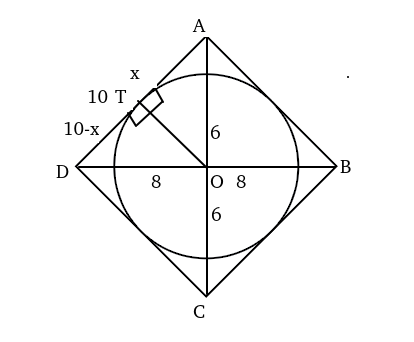Question 9

# A circle is inscribed in a rhombus with diagonals 12 cm and 16 cm. The ratio of the area of circle to the area of rhombus is

SolutionLet the length of radius be 'r'.

From the above diagram,

$$x^2+r^2=6^2\$$....(i)

$$\left(10-x\right)^2+r^2=8^2\$$----(ii)

Subtracting (i) from (ii), we get:

x=3.6 => $$r^2=36-\left(3.6\right)^2$$ ==> $$r^2=36-\left(3.6\right)^2\ =23.04$$.

Area of circle = $$\pi\ r^2=23.04\pi\$$

Area of rhombus= 1/2*d1*d2=1/2*12*16=96.

.'. Ratio of areas = 23.04$$\pi\$$/96=$$\frac{6\pi}{25}$$

### View Video Solution